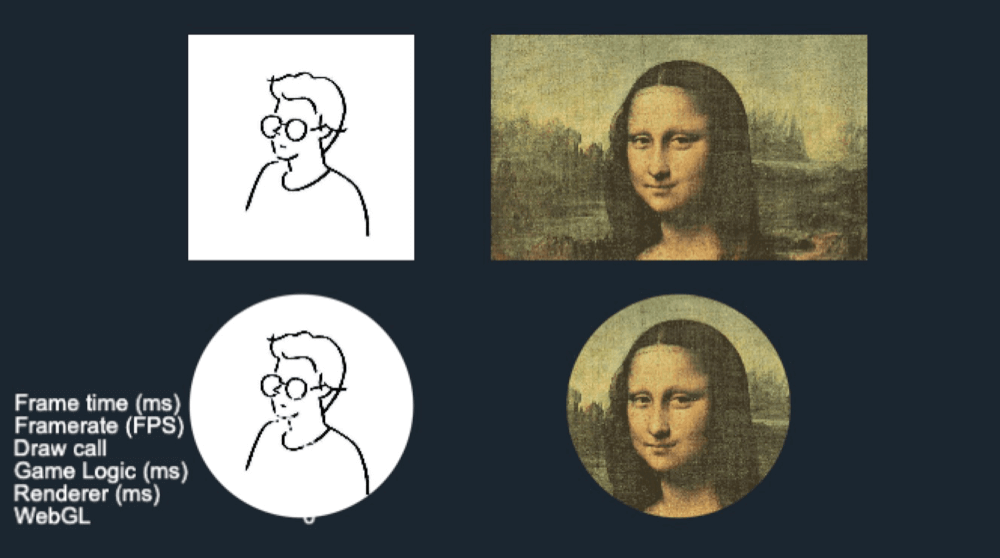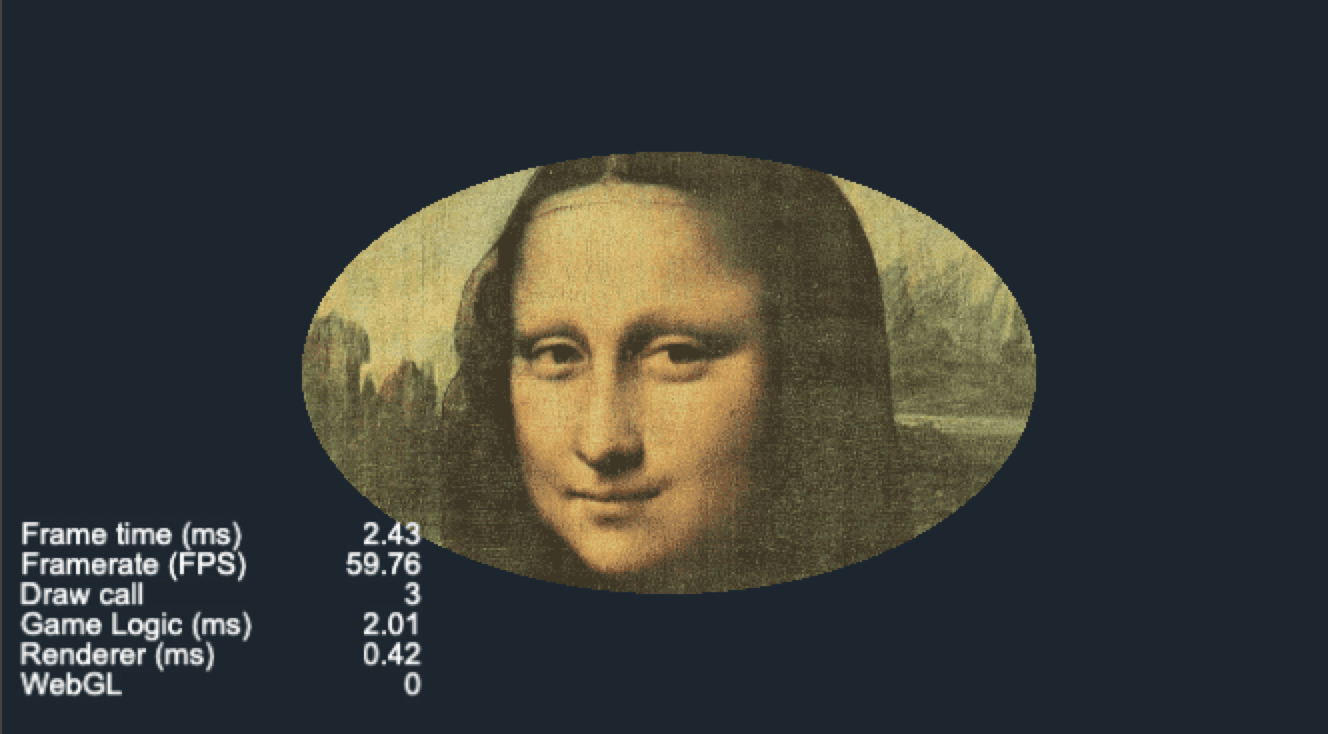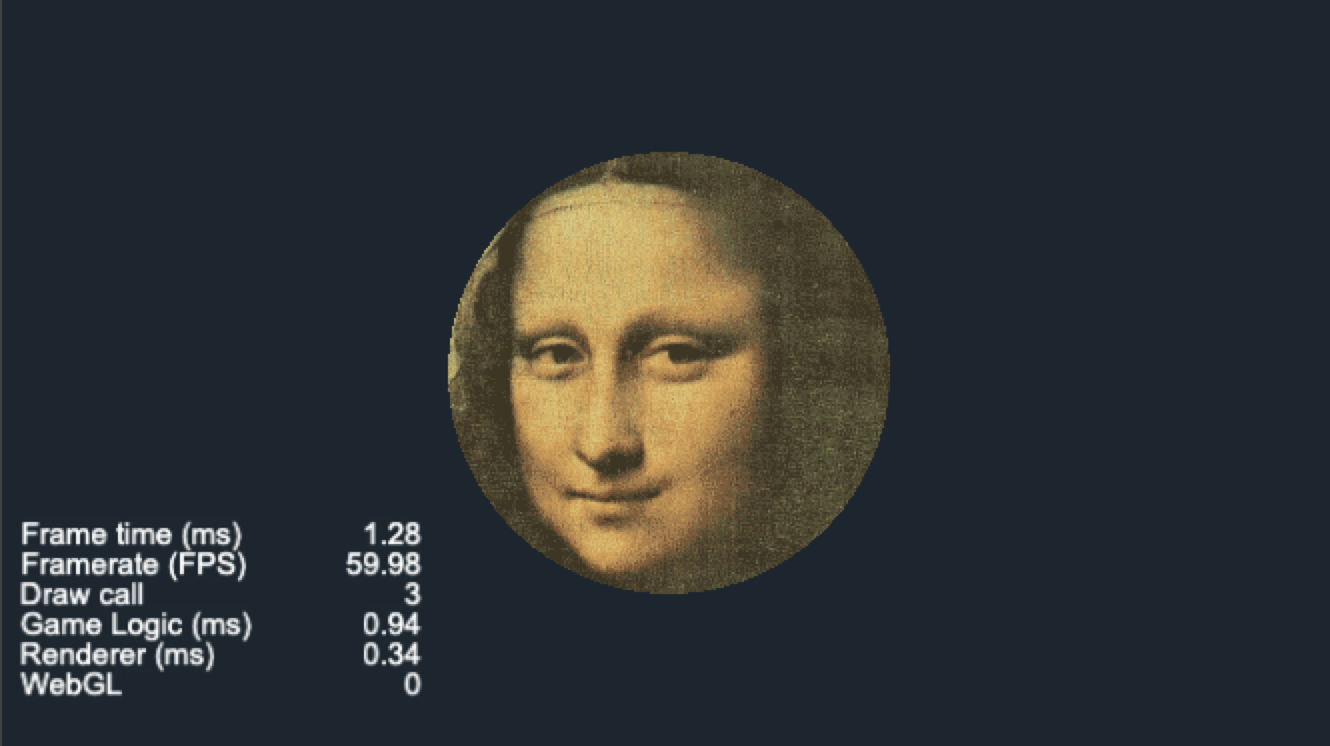# 效果演示# 实现思路

``````void main () {
vec4 color = vec4(1, 1, 1, 1);
color *= texture(texture, v_uv0);
color *= v_color;

color.a = step(length(v_uv0 - vec2(0.5,0.5)), 0.1);
gl_FragColor = color;
}
````````````void main () {
vec4 o = vec4(1, 1, 1, 1);
o *= texture(texture, v_uv0);
o *= v_color;

float rx = center.x * wh_ratio;
float ry = center.y;
float dis = (v_uv0.x * wh_ratio - rx) * (v_uv0.x * wh_ratio - rx) + (v_uv0.y  - ry) * (v_uv0.y - ry);

o.a = step(dis, 0.1);
gl_FragColor = o;
}
````````````void main () {
vec4 o = vec4(1, 1, 1, 1);
o *= texture(texture, v_uv0);
o *= v_color;

float rx = center.x * wh_ratio;
float ry = center.y;
float dis = (v_uv0.x * wh_ratio - rx) * (v_uv0.x * wh_ratio - rx) + (v_uv0.y  - ry) * (v_uv0.y - ry);

o.a = smoothstep(circle, circle - blur, dis);
gl_FragColor = o;
}
``````## 效果预览